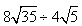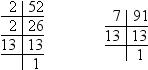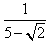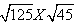# Test: Real Numbers

## 20 Questions MCQ Test Mathematics (Maths) Class 9 | Test: Real Numbers

Description
This mock test of Test: Real Numbers for Class 9 helps you for every Class 9 entrance exam. This contains 20 Multiple Choice Questions for Class 9 Test: Real Numbers (mcq) to study with solutions a complete question bank. The solved questions answers in this Test: Real Numbers quiz give you a good mix of easy questions and tough questions. Class 9 students definitely take this Test: Real Numbers exercise for a better result in the exam. You can find other Test: Real Numbers extra questions, long questions & short questions for Class 9 on EduRev as well by searching above.
QUESTION: 1

Solution:
QUESTION: 2

### The decimal fraction in which there are finite number of digits in its decimal part is called

Solution:

A decimal is a number with a decimal point. There are usually numbers to the left and numbers to the right of that decimal point. For example,​ 0.5 and 12.56 are both decimals. Decimals allow us to represent partial amounts of a whole, just like fractions. As a matter of fact, many decimals and fractions are interchangeable so either form can be used.

QUESTION: 3

### If two positive integers p and q can be expressed as p = ab2 and q = a3b; a, b being prime numbers, then LCM (p, q) is

Solution:

Given that, p = ab2 = a × b × b
and q = a3b = a × a × a × b
∴ LCM of p and q = LCM (ab2,a3b) = a × b × b × a × a = a3b2
[Since, LCM is the product of the greatest power of each prime factor involved in the numbers].

QUESTION: 4

If two positive integers a and b are written as a = x3y2 and b = xy3; x, y are prime numbers, then HCF (a, b) is

Solution:

Given that, a =x3y2 = x × x × x × y × y
and b = xy3 = x × y × y × y
∴ HCF of a and b = HCF (x3y2,xy3) = x × y × y = xy
[Since, HCF is the product of the smallest power of each common prime factor involved in the numbers]

QUESTION: 5

SimplifySolution:
QUESTION: 6

A forester wants to plant 44 apples tree, 66 banana trees and 110 mango trees in equal rows (in terms of number of trees). Also, he wants to make distinct rows of tree (i.e. only one type of tree in one row). The number of rows (minimum) that required is:

Solution:

In such case, we first need to find the HCF of 44, 66, 110.
44 = 2 x 2 x 11;
66 = 2 x 3 x 11;
110 = 2 x 5 x 11;
HCF = 2*11 = 22.
Then, the required numbers of rows,
= {(44/22)+(66/22)+(110/22)} = 10.

QUESTION: 7

The HCF and LCM of two numbers are 33 and 264 respectively. When the first number is completely divided by 2 the quotient is 33. The other number is:

Solution:

Given HCF = 33 and LCM = 264
First number a = 2(33) = 66
Let the second number be b
Recall the formula, LCM × HCF = a × b
264 × 33 = 66 × b
b =  264 × 33 / 66 = 132
The second number is 132.

QUESTION: 8

What will be the greatest possible length of the planks, if three pieces of timber 42 m, 49 m and 63 m long have to be divided into planks of the same length?

Solution:

The lengths of three pieces of timber are 42m, 49m and 63m respectively.

We have to divide the timber into equal length of planks.

∴ Greatest possible length of each plank = HCF (42, 49, 63)

Prime factorization:

42 = 2 × 3 × 7

49 = 7 × 7

63 = 3 × 3 × 7

∴HCF = Product of the smallest power of each common prime factor involved in the numbers = 7

Hence, the greatest possible length of each plank is 7m.

QUESTION: 9

If the HCF of 65 and 117 is expressible in the form 65m – 117, then the value of m is

Solution:

Find the HCF of 65 and 117,

117 = 1×65 + 52

65 = 1× 52 + 13

52 = 4 ×13 + 0

∴ HCF of 65 and 117 is 13.

65m - 117 = 13

65m = 117+13 = 130

∴m =130/65 = 2

QUESTION: 10

The least number that is divisible by all the numbers from 1 to 10 (both inclusive) is

Solution:

Factors of 1 to 10 numbers
1=1
2=1×2
3=1×3
4=1×2×2
5=1×5
6=1×2×3
7=1×7
8=1×2×2×2
9=1×3×3
10=1×2×5
∴ LCM of number 1 to 10 = LCM (1,2,3,4,5,6,7,8,9,10)
= 1×2×2×2×3×3×5×7 = 2520

QUESTION: 11

What is the greatest possible speed at which a man can walk 52 km and 91 km in an exact number of minutes?

Solution:

Factors of 52 and 91 areso 52 = 2 × 2 × 13

and 91 = 7 × 13

∴ H.C.F. of 52 and 91 = 13

Hence required speed in minutes = 13 km / min.

QUESTION: 12

Pairs of natural numbers whose least common multiple is 78 and the greatest common divisor is 13 are:

Solution:

Let the no.s be a & b,

GCD [ a , b ] = 13;
=> Let a = 13m and b = 13n

Now, LCM [ a , b ] = 78
=> 13m | 78
=> m | 6 and similarly, n | 6; --> Also, m does not divide n

.'. Possible values of (m,n) are :->
--> [ 1 , 6 ] , [ 2 , 3 ]
.'. Possible values for ( a , b ) are :-> [ 13 , 78 ] , [ 26 , 39 ]

QUESTION: 13

Which of the following is a rational number?

Solution:

0 is an integer and all integers are rational numbers.

QUESTION: 14

If two positive integers p and q can be expressed as p = ab2 and q = a3b; a, b being prime numbers, then LCM (p, q) is

Solution:

As per question, we have,
p = ab2 = a × b × b
q = a3b = a × a × a × b
So, their Least Common Multiple (LCM) = a3 × b2

QUESTION: 15

Every real number is

Solution:

Either you can make a fraction from two whole numbers (denominator ≠ 0), thus a rational number. Or you can't, thus irrational.

QUESTION: 16

The largest number which divides 70 and 125, leaving remainders 5 and 8 respectively, is

Solution:

Since, 5 and 8 are the remainders of 70 and 125, respectively. Thus, after subtracting these remainders from the numbers, we have the numbers 65 = (70-5),
117 = (125 – 8), which is divisible by the required number.
Now, required number = HCF of 65,117                                     [for the largest number]
For this, 117 = 65 × 1 + 52 [∵ dividend = divisior × quotient + remainder]
⇒ 65 = 52 × 1 + 13
⇒ 52 = 13 × 4 + 0
∴ HCF = 13
Hence, 13 is the largest number which divides 70 and 125, leaving remainders 5 amnd 8.

QUESTION: 17

Rationalizing Factor isis

Solution:
QUESTION: 18

The least number that is divisible by all the numbers from 1 to 10 (both inclusive) is

Solution:

We have to find the least number that is divisible by all numbers from 1 to 10.

∴ LCM (1 to 10) = 23 × 32 × 5 × 7 = 2520

Thus, 2520 is the least number that is divisible by every element and is equal to the least common multiple.

QUESTION: 19

SimplifySolution:

√5×√5×√5 × √5×√3×√3 5×5×3 =75

QUESTION: 20

The least number that is divisible by all the numbers from 1 to 10 (both inclusive) is

Solution:

Factors of 1 to 10 numbers
1=1
2=1×2
3=1×3
4=1×2×2
5=1×5
6=1×2×3
7=1×7
8=1×2×2×2
9=1×3×3
10=1×2×5
∴ LCM of number 1 to 10 = LCM (1,2,3,4,5,6,7,8,9,10)
= 1×2×2×2×3×3×5×7 = 2520